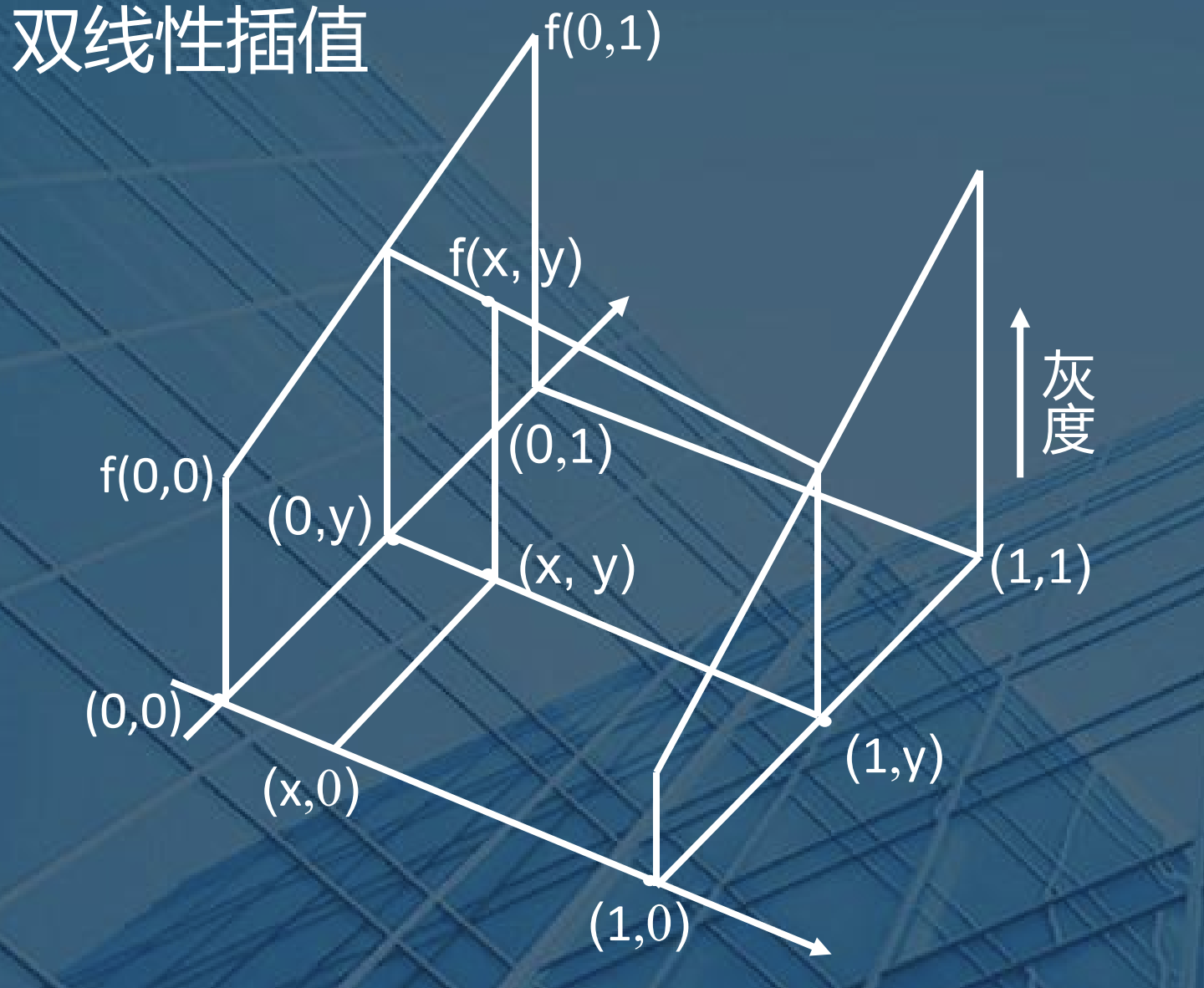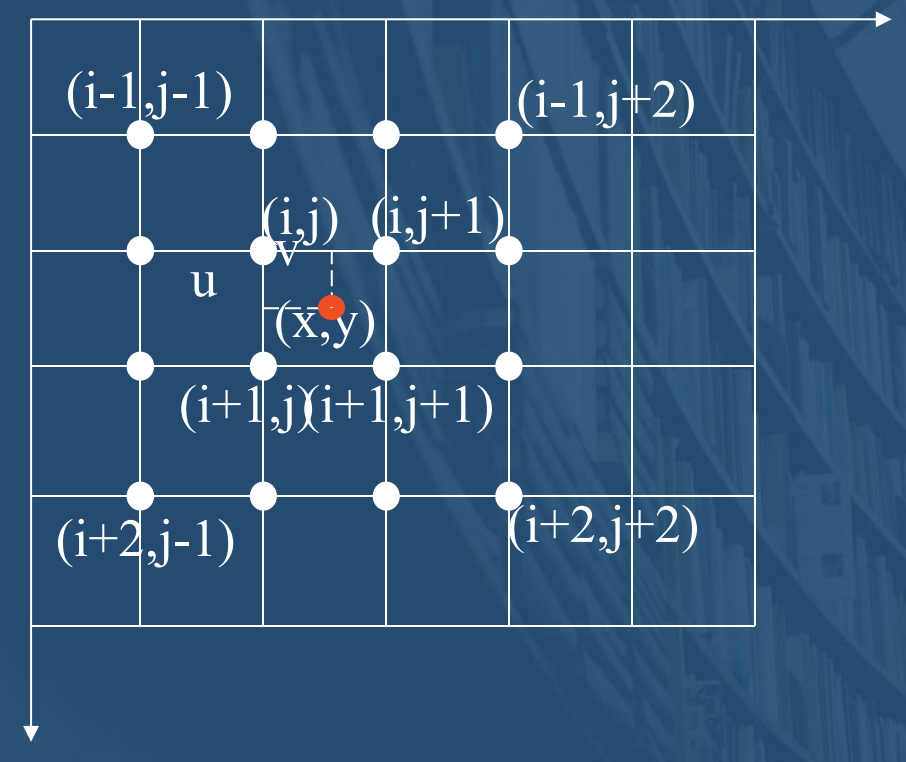## 最邻近插值

$$f(x)=f(x_{k})​$$

$$\frac {1}{2} (x_{k-1}+x_{k})<x< \frac {1}{2}(x_{k}+x_{k+1})$$

(x为浮点数,$x_{k}$是离x最近的整数点的灰度值)

## 双线性插值

$$f(x,y)=ax+by+cxy+d$$

$a=f(1,0)-f(0,0)$,

$b=f(0,1)-f(0,0)$,

$c=f(1,1)+f(0,0)-f(0,1)-f(1,0)$,

$d=f(0,0)$## 双三次差值

$$f(x,y)=\sum \limits_{i=0}^3 \sum \limits_{i=0}^3 a_{ij}x^i y^j$$